### IMO Shortlist 2015 problem N6

Kvaliteta:
Avg: 0.0
Težina:
Avg: 8.0

Let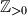$\mathbb{Z}_{>0}$ denote the set of positive integers. Consider a function$f: \mathbb{Z}_{>0} \to \mathbb{Z}_{>0}$. For any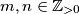$m, n \in \mathbb{Z}_{>0}$ we write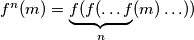$f^n(m) = \underbrace{f(f(\ldots f}_{n}(m)\ldots))$. Suppose that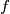$f$ has the following two properties:

(i) if$m, n \in \mathbb{Z}_{>0}$, then$\frac{f^n(m) - m}{n} \in \mathbb{Z}_{>0}$;

(ii) The set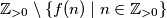$\mathbb{Z}_{>0} \setminus \{f(n) \mid n\in \mathbb{Z}_{>0}\}$ is finite.

Prove that the sequence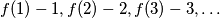$f(1) - 1, f(2) - 2, f(3) - 3, \ldots$ is periodic.

(Singapore)

Source: https://www.imo-official.org/problems/IMO2015SL.pdf